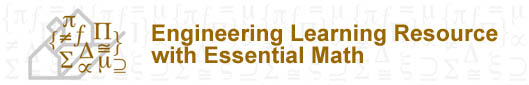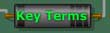Learning Resource Topics Overview 1 Charge & Coulomb’s Law 2 Electric Current 3 Voltage 4 Resistance 5 Ohm’s Law 6 Power & Energy 7 Elements of Electric Circuits 8 Kirchhoff’s Law 9 Series Circuits 10 Parallel Circuits 11 Analysis of Combination Circuits 12 Capacitance 13 Inductance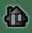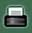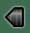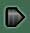# Integral Voltage-Current Relationship

The capacitance may be defined by the voltage-current relationship:The ideal capacitor defined by the above equation is a mathematical model of a real device. The above equation provides several important characteristics. A time-constant voltage across a capacitor results in zero current passing through it; a capacitor is therefore an open circuit to DC.

The capacitor voltage may be expressed in terms of the current by integrating the above equation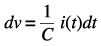Now, we integrate the above Equation between the times t0 and t1 and between the corresponding voltages v( t0) and v( t1)(12.8)

The above equation may be written as an indefinite integral plus a constant of integration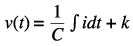(12.9)

where the constant of integration depends on the initial conditions (t = 0). The power delivered to the capacitor is(12.10)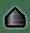Content and Pedagogy© 2004, University of Ottawa, Faculty of Engineering and Faculty of Education
Design and Production © 2004, University of Ottawa, Centre for e-Learning